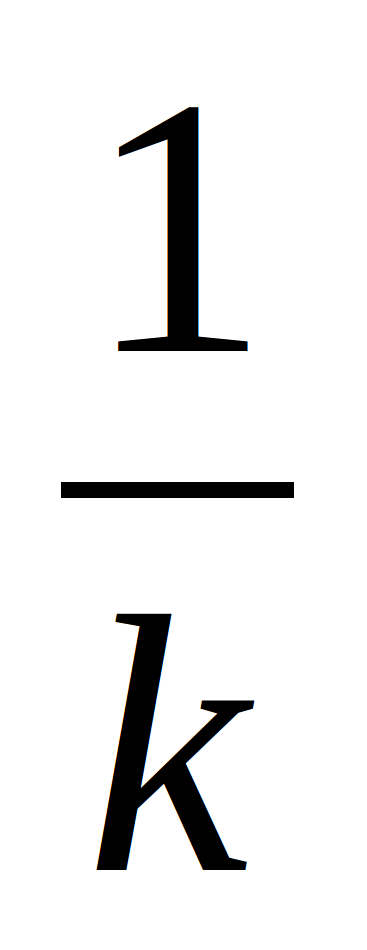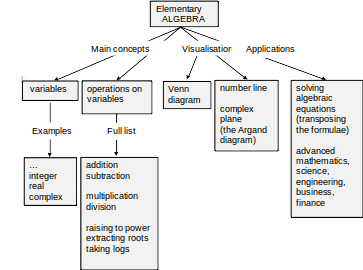Elementary Functions and Complex Numbers Made Simple

……………………………………………………

14 September 2013

I. INTRODUCTION

II. CONCEPT MAPS

III. LECTURES

Lecture 4. FUNCTIONS

4.1 Variables

4.2 Functions

4.3 Elementary operations on functions

4.4 Order of Operations (extended)

4.5 Applications of real functions of real variable and operations on such functions

4.6 A historical note

4.7 Instructions for self-study

Lecture 5. Real FUNCTIONS of One Real Variable: Graphs, Polynomials

5.1 Graphical representation of real functions of one real variable

5.2 How to use graphs of real functions y = f(x) of one real variable

5.3 Applications of graphs

5.4 Elementary functions: monomials (natural powers) and polynomials

5.5 Instructions for self-study

Lecture 6. Real FUNCTIONS of One Real Variable: Exponential Functions, Logarithmic Functions, Inverse Functions

6.1 Exponential functions

6.2 Logarithmic functions

6.3 Revision: Trigonometry

6.4 A historical note

6.5 Instructions of self-study

Lecture 7. Real FUNCTIONS of One Real Variable: Trigonometric Functions, Inverse Trigonometric Functions, Hyperbolic Functions

7.1 Trigonometric functions

7.2 Inverse trigonometric functions

7.3 Hyperbolic functions and their inverses

7.4 Instructions for self-study

Lecture 8. Real FUNCTIONS of One Real Variable: Sketching and Using Graphs, Simple Transformations

8.1 Sketching graphs using a table

8.2 Using graphs to find y given x

8.3 Using graphs to find x given y

8.4 Sketching graphs using simple transformations

8.5 Sketching graphs using several simple transformations

8.6 Completing the square

8.7 Applications of simple transformations

8.8 Instructions for self-study

Lecture 9. Real FUNCTIONS of One Real Variable: Sketching Graphs by Simple Transformations (ctd.)

9.1 Sketching graphs using several simple transformations (ctd.)

9.2 Sketching graphs using pointwise operations

9.3 Instructions for self-study

Lecture 10. ALGEBRA: Addition of Complex Numbers, the Argand Diagram, Forms of Complex Numbers

10.1 Imaginary unity j

10.2 Applications of complex numbers: solving quadratic equations

10.3 Operations: the whole powers of j

10.4 Variables: definition of a complex number z

10.5 Operations: addition of complex numbers z1 and z2

10.6 Operations: subtraction of complex numbers

10.7 The Argand diagram and Cartesian form of a complex number

10.8 The Argand diagram and polar form of a complex number

10.9 The Cartesian-polar and polar-Cartesian transformations

10.10 The trigonometric form of a complex number

10.11 Euler’s formula

10.12 The exponential form of a complex number

10.13 Applications

10.14 A historical note

10.15 Instructions for self-study

Lecture 11. ALGEBRA: Multiplication and Division of Complex Numbers

11.1 Multiplication of a complex number z by a real number

11.2 Operations: multiplication of complex numbers z1 and z2

11.3 Operations: complex conjugate z* of a complex number z

11.4 Operations: division of complex numbers z1 and z2

11.5 Integer powers of complex numbers

11.6 The fractional powers(k-th roots) of complex numbers

11.7 Instructions for self-study

Lecture 12. ALGEBRA: Fractional Powers, Logs and Loci of Complex Numbers

12.1 The fractional powers(whole roots k) of complex numbers (ctd.)

12.2 Logs of complex numbers

12.3 Loci on the Argand diagram

12.4 Applications

12.5 Instructions for self-study

IV. SUMMARIES

Algebra Summary

Functions Summary

Order of Operations Summary

Trigonometry Summary

Complex Numbers

Decision Tree For Solving Simple Equations

V. GLOSSARY

VI. STUDY SKILLS FOR MATHS

VII. TEACHING METHODOLOGY (FAQs)

1. # INTRODUCTION

These notes are based on the lectures delivered by the author to engineering students of London South Bank University over the period of 16 years. This is a University of widening participation, with students coming from many different countries, many of them not native English speakers. Most students have limited mathematical background and limited time both to revise the basics and to study new material. A system has been developed to assure efficient learning even under these challenging restrictions. The emphasis is on systematic presentation and explanation of basic abstract concepts. The technical jargon is reduced to the bare minimum.

Nothing gives a teacher a greater satisfaction than seeing a spark of understanding in the students’ eyes and genuine pride and pleasure that follows such understanding. The author’s belief that most people are capable of succeeding in - and therefore enjoying - the kind of mathematics that is taught at Universities has been confirmed many times by these subjective signs of success as well as genuine improvement in students’ exam pass rates. Interestingly, no correlation had ever been found at the Department where the author worked between the students’ qualification on entry and graduation.

The book owns a lot to the authors’ students – too numerous to be named here - who talked to her at length about their difficulties and successes, e.g. see Appendix VII on Teaching Methodology. One former student has to be mentioned though – Richard Lunt – who put a lot of effort into making this book much more attractive than it would have been otherwise.

The author can be contacted through her website www.soundmathematics.com. All comments are welcome and teachers can obtain there the copy of notes with answers to questions suggested in the text as well as detailed Solutions to suggested Exercises. The teachers can then discuss those with students at the time of their convenience.

Good luck everyone!

1. # CONCEPT MAPS

Throughout when we first introduce a new concept (a technical word or phrase) or make a conceptual point we use the bold red font. We use the bold blue to verbalise or emphasise an important idea.

Two major topics are covered in these Notes, Functions and Complex Numbers. You can understand these topics best if you first study the Lecture Notes 1 – 3 on Elementary Algebra. Here is a concept map of Elementary Algebra.